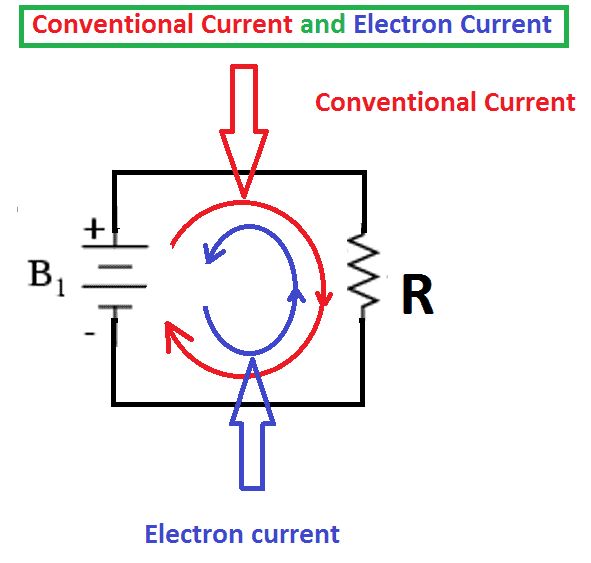# What is Conventional Current and Electron Current

## What is conventional current and electron current:

Two Types of currents are normally considered in circuits

• Conventional Current
• Electron Current

## Electron Current:

Normally, Flow of electron or flow of charge is called current. Two types of current are normally employed is electric circuit one is Conventional current and another one is Electron current. The flow of electrons from source negative terminal to positive terminal is termed as electron current. Here all Electrons flow from the negative terminal to the positive.

Since electrons, the charge carriers in metal wires and most other parts of electric circuits, have a negative charge, as a consequence, they flow in the opposite direction of conventional current flow in an electrical circuit.

## Conventional current

A flow of positive charges offers a similar current, and has a similar result in an electrical circuit, as an equal flow of negative charges within the opposite direction of electron current. Since current is often the flow of either positive or negative charges or both, a convention is required for the direction of current independent of the kind of charge carriers. The direction of conventional current is always same as the direction of the positive charges.

Conventional current is nothing but a flow of positive charge carriers causes current to flow. Conventional current flows from the positive terminal to the negative. Here the current flow due to positive charges. Positive charges flow from positive terminal to negative terminal. In general, analyzing an electrical circuit yields results that are independent of the assumed direction of current flow. Conventional current flow is the standard that almost all of the world follows to solve a circuit problem.

## Conventional current and electron current Identification

Consider Circuit diagram with voltage source as battery and the resistance R is connected with a voltage source. Here the there is a closed path electron starts flow from batter’s negative terminal to positive terminal. The current flowing from battery’s negative terminal to positive terminal is called electron current. anyhow to analyze a circuit we assume the current flows from positive terminal to negative terminal of the battery. This current is called conventional current.